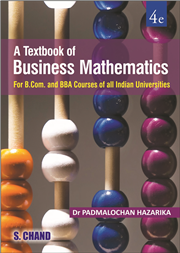Home > Higher Education > Mathematics > A TEXTBOOK OF BUSINESS MATHEMATICS# A TEXTBOOK OF BUSINESS MATHEMATICS, 4/e

 ISBN : 9789352533121 Pages : 736 Binding : Paperback Language : English Imprint : S. Chand Publishing Trim size : 6.75" x 9.5" inches Weight : 1.00kg © year : 2016
 List Price :525.00
 Offer Price :420.00

20% OFF

# In Stock

Credit Card / Debit Card / Internet BankingThe new edition of A Textbook of Business Mathematics inches on its earlier editions and continues to provide a comprehensive coverage of important topics and concepts in business mathematics. The text integrates the standard curriculum and the manifold requirements of undergraduate business maths students. The book is carefully written and structured to simplify business maths and equips students with the knowledge and practice they need to fully learn each concept. Abundant solved examples and exercises incorporated in the text help in effective learning process and examination preparation for students. • Incorporation of important techniques of Operations Research such as transportation and assignment problem  • More than 750 solved examples and exercises with solutions for extensive practice • Objective-type questions to test learning - a distinctive new feature of this edition Unit-I: Algebra • Revision of Basic Algebra and Commercial Arithmetic • Indices and Logarithms • Simultaneous Linear Equations and Quadratic Equations • Arithmetic Progression (A.P.), Geometric Progression (G.P.) and Harmonic Progression (H.P.) • Permutation and Combination • Binomial Theorem • Set Theory • Determinants • Vectors and Matrices Unit-II: Mathematics of Finance • Simple Interest and Compound Interest • Annuity Unit-III: Coordinate Geometry of Two Dimensions • Rectangular Cartesian Coordinates in a Plane • Equations of Straight Lines • Equations of Non-Linear Curves: Circle and Parabola Unit-IV: Calculus • Functions, Limits and Continuity of Functions • Derivative or Differential Coefficient • Application of Derivative in Economics and Commerce • Maxima and Minima • Partial Derivatives and Homogeneous Functions • Integration as Antiderivative • Definite Integral and Application of Integration in Business and Economics Unit-V: Certain Techniques of Operations Research • Introduction to Operations Research (OR) • Linear Programming • Basic Concepts of Transportation Problem and Assignment Problem • Theory of Games### More Books By Author#### A Textbook of Business ...

9788121924566350.00280.00

20% OFF525.00420.00